• 对RLC串联电路的二阶时域微分方程和复频域代数方程的数学模型进行详细分析，并运用Proteus软件建立其电路模型，研究RLC串联二阶电路系统在方波激励信号作用下临界阻尼、欠阻尼、过阻尼情况的状态响应.通过图表分析和...
• 二阶系统的数学模型2. 二阶系统地单位阶跃响应2.1 无阻尼2.2. 欠阻尼 1. 二阶系统的数学模型 传递函数： 为了使研究结果具有普遍意义，二阶系统的传递函数写为以下标准形式： ωn\omega_{n}ωn​：称为无阻尼...


2020-05-26-二阶系统时域分析

文章目录1. 二阶系统的数学模型2. 二阶系统地单位阶跃响应2.1 无阻尼2.2. 欠阻尼2.3 临界阻尼2.4 小结3. 欠阻尼二阶系统的动态过程分析3.1 上升时间$t_{r}$3.2 峰值时间$t_{p}$3.3 超调量$\sigma\%$3.4 调节时间$t_{s}$
1. 二阶系统的数学模型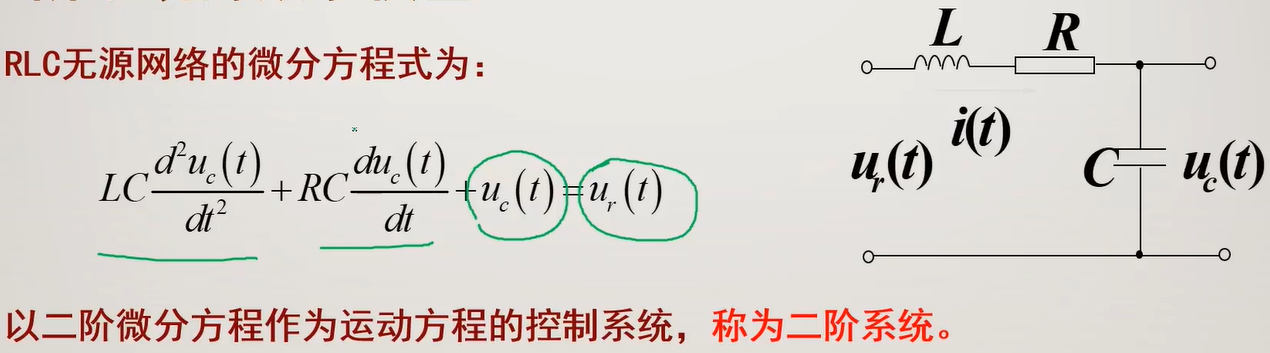传递函数：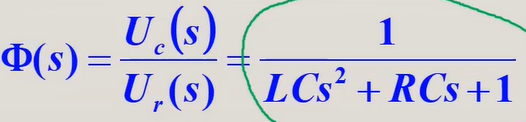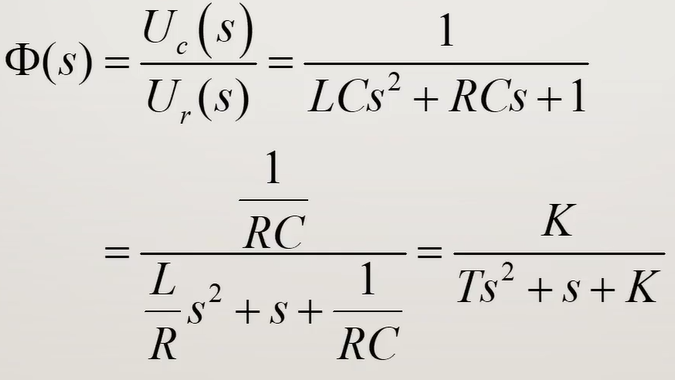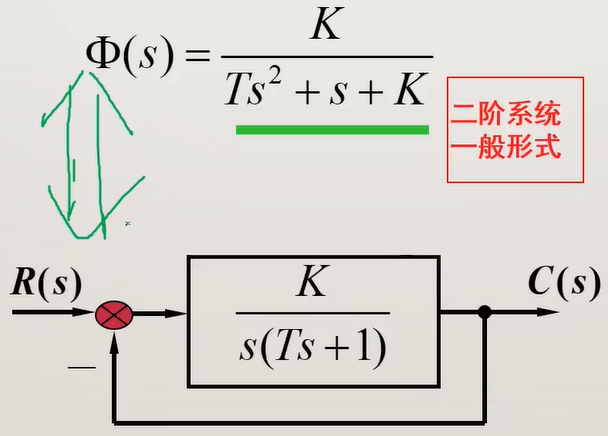为了使研究结果具有普遍意义，二阶系统的传递函数写为以下标准形式：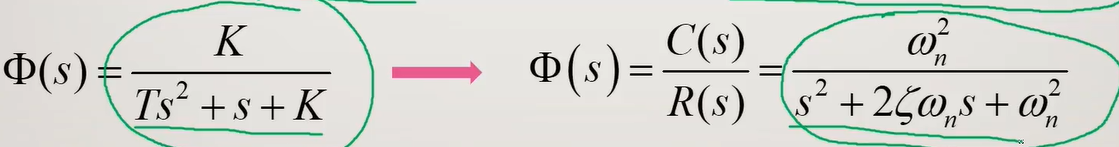$\omega_{n}$：称为无阻尼自然振荡角频率，简称自然频率。
$\omega_{n}=\sqrt{\frac{K}{T}}$
$\zeta$：称为阻尼比，或相对阻尼系统。
$\zeta=\frac{1}{2\sqrt{KT}}$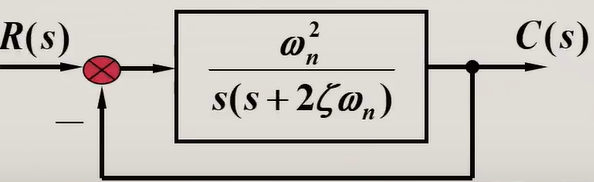问：极点和零点哪个更本质地影响系统的输出响应？
答：极点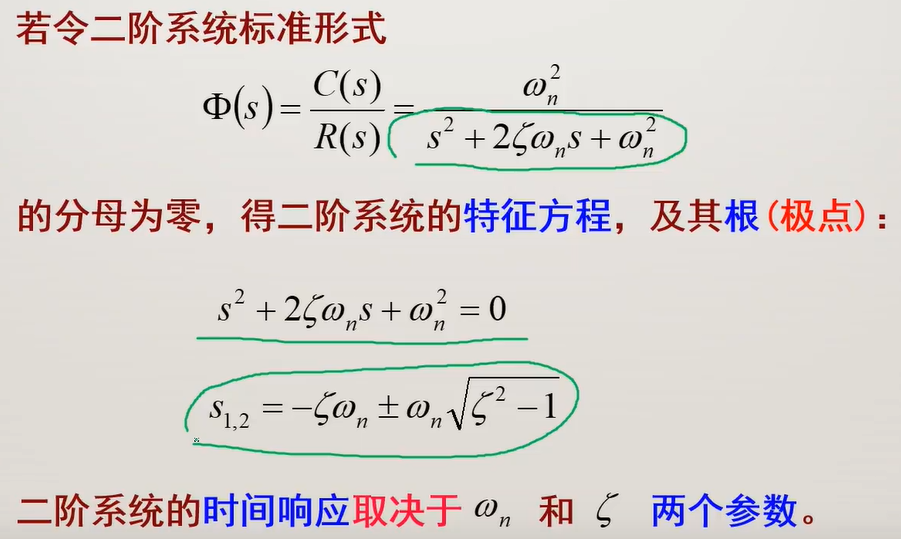问：你认为两个参数$\zeta,\omega_{n}$，哪个可以更本质地影响系统输出？
答：$\zeta$。

2. 二阶系统地单位阶跃响应
$s_{1,2}=-\zeta\omega_{n} \pm \omega_{n} \sqrt{\zeta^2-1}$
(1) 无阻尼
$\zeta=0$
(2) 欠阻尼
$0<\zeta<1$
(3) 临界阻尼
$\zeta=1$
(4) 过阻尼
$\zeta>1$
(5) 负阻尼
$-1<\zeta<0 \\ \zeta=-1\\ \zeta<-1$
2.1 无阻尼
$\zeta=0\\ s_{1,2}=\pm j\omega_{n}$

问：既然无阻尼情况的特征根是两个纯虚根，请问系统的输出呈现何种形式？
答：等幅振荡。

2.2. 欠阻尼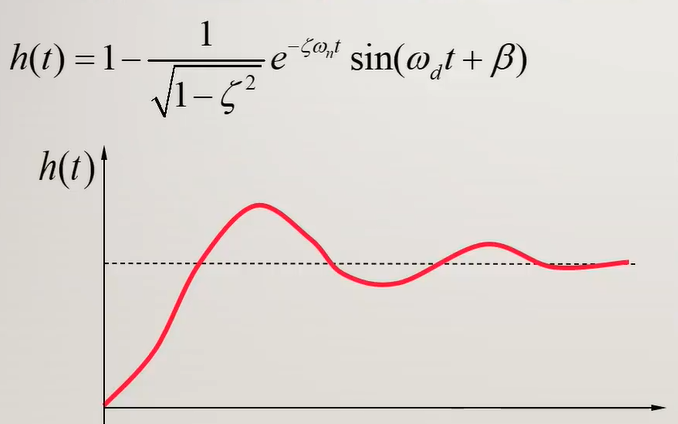$0<\zeta<1\\ s_{1,2}=-\zeta \omega_{n} \pm \omega_{n}\sqrt{\zeta^2-1}\\ 变为\\ s_{1,2}=-\zeta \omega_{n} \pm j\omega_{n}\sqrt{1-\zeta^2}$

问：既然欠阻尼情况有两个共轭复根，且均具有负实部，请问输出呈现何种形式？
答：振荡收敛。

衰减速度取决于衰减系数，越大，收敛越快。
$\sigma=\zeta \omega_{n}$
振荡频率
$\omega_{d}=\omega_{n} \sqrt{1-\zeta^2}$
$\zeta$增大，$\omega_{d}$减小，$\zeta \omega_{n}$增大，振荡频率减小，衰减速度变快，振荡减弱。

2.3 临界阻尼
$\zeta=1\\ s_{1,2}=-\zeta \omega_{n} \pm \omega_{n} \sqrt{\zeta^2-1}\\ 变为\\ s_{1,2}=-\omega_{n}$

既然临界阻尼情况有两个负实根，请问输出呈现何种形式？
答：等幅振荡。

在临界阻尼情况下，系统的单位阶跃响应为：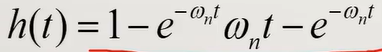响应导数：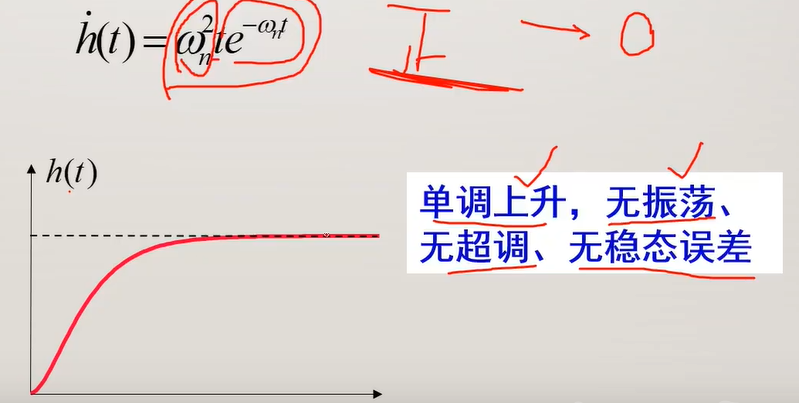问：请问临界阻尼中的“临界”，指的是哪两种情况的临界？
答：

稳定与不稳定的临界
振荡与不振荡的临界
实根与虚根的临界
左半平面与右半平面的临界

2.4 小结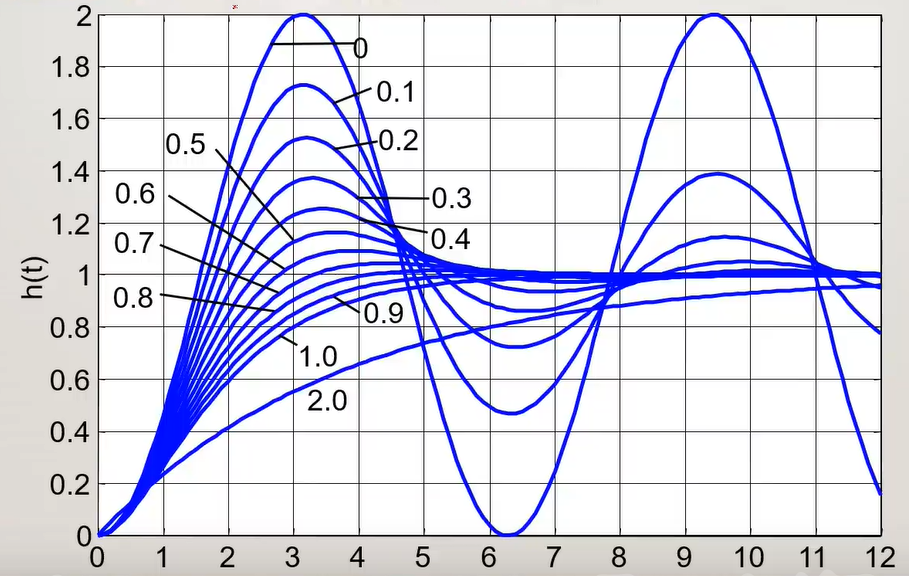问：思考过阻尼($\zeta>1$)情况的根具有何种形式，并选择输出呈现何种形式？
答：单调收敛，因为具有两个负实根$s_{1,2}=-\zeta\omega_{n}\pm\omega_{n}\sqrt{\zeta^2-1}$.

问：思考过阻尼($-1<\zeta<0$)情况的根具有何种形式，并选择输出呈现何种形式？
答：振荡发散。因为具有正实部和虚部。

3. 欠阻尼二阶系统的动态过程分析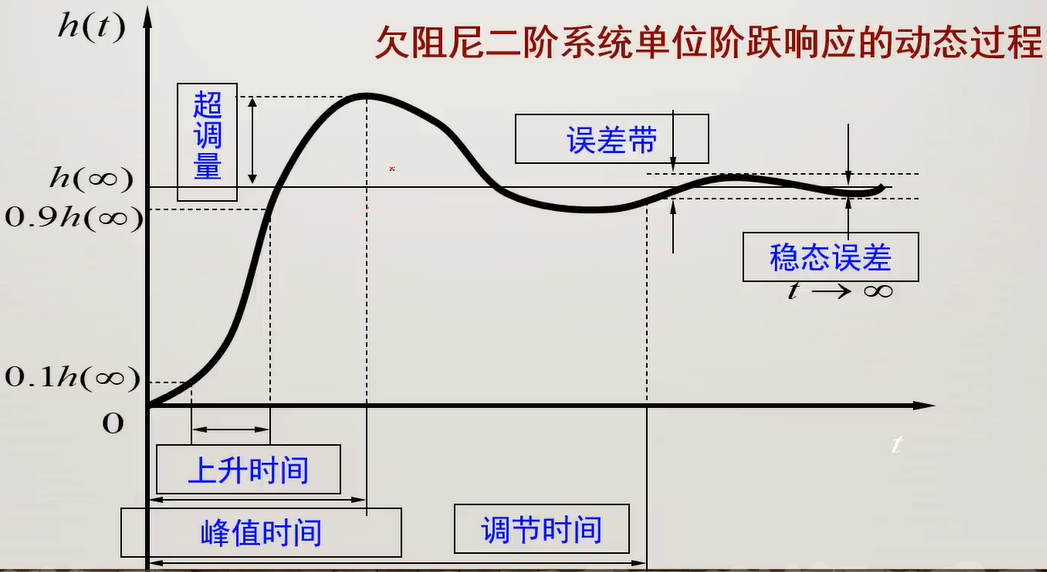问：上升时间有两种定义，一种是10%终值到90%终值的时间，一种是0到终值的时间，请问对于二阶欠阻尼系统的输出，应该用哪一种？
答：0到终值的时间。
问：误差带有两种定义，是哪两种？
答：2%和5%

3.1 上升时间$t_{r}$
$t_{r}=\frac{\pi-\beta}{\omega_{d}}\\ 阻尼振荡频率：\omega_{d}=\omega_{n} \sqrt{1-\zeta^2}\\ 阻尼角：\beta=arctan(\frac{\sqrt{1-\zeta^2}}{\zeta})$
3.2 峰值时间$t_{p}$
$t_{p}=\frac{\pi}{\omega_{d}}$
3.3 超调量$\sigma\%$
$\sigma\%=e^{-\frac{\zeta\pi}{\sqrt{1-\zeta^2}}} \times100\%$

超调量仅是阻尼比$\zeta$的函数，与自然频率$\omega_{n}$无关。
超调量与阻尼比关系曲线，阻尼比越大，超调量越小。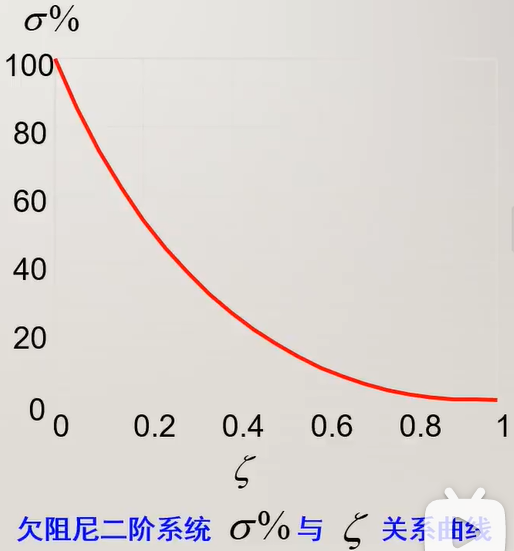一般，选取
$\zeta=0.4到0.8\\ \sigma\%=1.5\%到25.4\%$

3.4 调节时间$t_{s}$
$t_{s}\approx \frac{4.4}{\zeta \omega_{n}} (\Delta=0.02)$
$t_{s}\approx \frac{3.5}{\zeta \omega_{n}} (\Delta=0.05)$

下一篇：静态误差系数法


展开全文自动控制原理 笔记
• 一、二阶系统的电子模拟及阶跃响应的动态分析一、实验目的1．学习典型环节的电子模拟方法及在电子模拟器上建立数学模型的方法。2．学习时域响应的测试方法，树立时域的概念。3．明确一、二阶系统的阶跃响应及其性能...
一、二阶系统的电子模拟及阶跃响应的动态分析一、实验目的1．学习典型环节的电子模拟方法及在电子模拟器上建立数学模型的方法。2．学习时域响应的测试方法，树立时域的概念。3．明确一、二阶系统的阶跃响应及其性能域结构参数的关系。二、实验内容1．建立一阶系统的电子模型，观察并测量不同时间常数T的阶跃响应及性能指标调节时间ts。2．建立二阶系统的电子模型，观察并测量不同阻尼比ξ时的阶跃响应及性能指标调节时间ts超调量δ%。三、实验的原理与方法1．一阶系统微分方程(Ts+1)Uc-Ur传递函数其模拟运算电路如下图所示。由图所示取R1=R2则K=1，Ts=R2C选取不同的电阻值，使T分别为0.1s、0.2s、0.5s、1s时，观测并记录阶跃响应，计算调节时间ts。2．二阶系统传递函数当ωn=1(rad/s)时，系统的动态结构如下图(b)所示。根据动态结构图画出模拟运算电路下图。若取R2C2=1，R3C3=1则为观测不同阻尼比对二阶系统的影响，可以选配不同的电阻电容值使阻尼比ξ分别为0.1、0.5、0.7、1。观察并记录响应曲线、测量H向应性能指标调节时问ts、超调量σ%。四、实验设备及元器件电子模拟器一台超低频双线长余辉示波器一台双线笔录仪一台(非必备设备)直流稳压电源一台三用表一台元器件 电容 1μF 2.2μF 4.7μF 6.8μF 10μF可变电阻 100kΩ 470kΩ接插件导线接线柱鱼形夹等
展开全文• 目 二阶系统模糊控制算法的研究 专 业 电气工程及其自动化 姓 名 指 导 教 师 学 期 2015-2016 二阶系统的模糊控制算法的研究 学生 指导老师 : 摘 要: 模糊控制是以模糊数学为基础发展的为一些无法建立数学模型或者...
• 课 程 题 目 二阶系统模糊控制算法研究 专 业 电气工程及其自动化 姓 名 指 导 教 师 学 期 20XX-20XX 二阶系统模糊控制算法研究 学生 指导老师: 摘 要:模糊控制是以模糊数学为基础发展为部分无法建立数学模型数学...
• 自动控制理论（2）——控制系统的数学模型（微分方程、传递函数 自动控制理论（3）——控制系统的数学模型（系统框图和信号流图 自动控制理论（4）——系统的时域性能指标和一阶系统的时域分析 文章目录系列文章...
系列文章目录
自动控制理论（1）——自动控制理论概述
自动控制理论（2）——控制系统的数学模型（微分方程、传递函数
自动控制理论（3）——控制系统的数学模型（系统框图和信号流图
自动控制理论（4）——系统的时域性能指标和一阶系统的时域分析

文章目录系列文章目录一、二阶系统数学模型二、二阶系统单位阶跃响应1.$\ ζ=0$(零阻尼)2.$\ ζ>1$( 过阻尼)3.$\ ζ=1$( 临界阻尼)4.$\ 0<ζ<1$( 欠阻尼)5.总结三 、欠阻尼二阶系统性能指标计算1.上升时间$\ t_r$2.峰值时间$\ t_p$3.超调量$\ σ\%$4.调节时间$\ t_s$5.延迟时间$\ t_d$四 、具有零点的二阶系统分析

一、二阶系统数学模型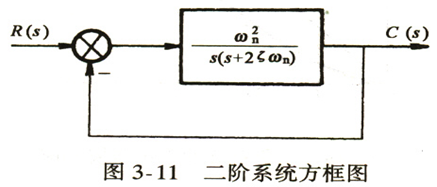开环$\ G(s)=\frac{ω_n^2}{s(s+2ζω_n)}$
闭环$\ ϕ(s)=\frac{ω_n^2}{s^2+2ζω_n s+ω_n^2 }$
特征方程$\ s^2+2ζω_n s+ω_n^2=0$
$\ ζ$——阻尼比
$\ ω_n$——无阻尼自然振荡角频率
$\ σ=ζω_n$——衰减系数
$\ ω_d=ω_n √(1-ζ^2 )$——系统的阻尼振荡角频率
二、二阶系统单位阶跃响应
1.$\ ζ=0$(零阻尼)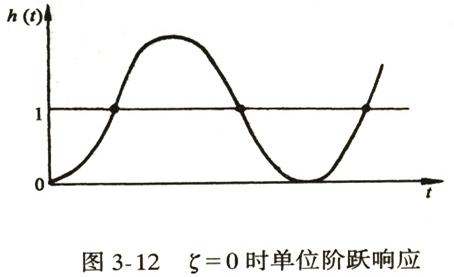响应曲线为等幅振荡曲线。
2.$\ ζ>1$( 过阻尼)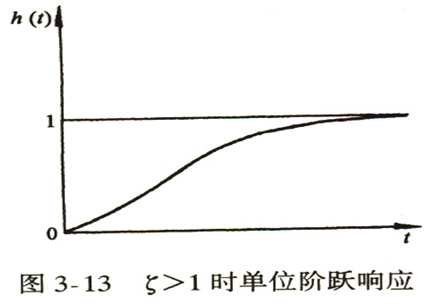两个不等负实根
3.$\ ζ=1$( 临界阻尼)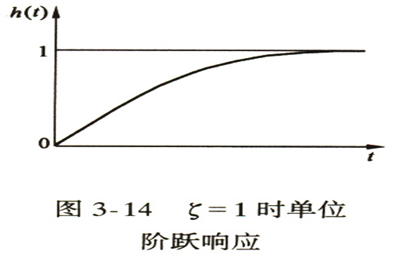两个相等的负实根
4.$\ 0<ζ<1$( 欠阻尼)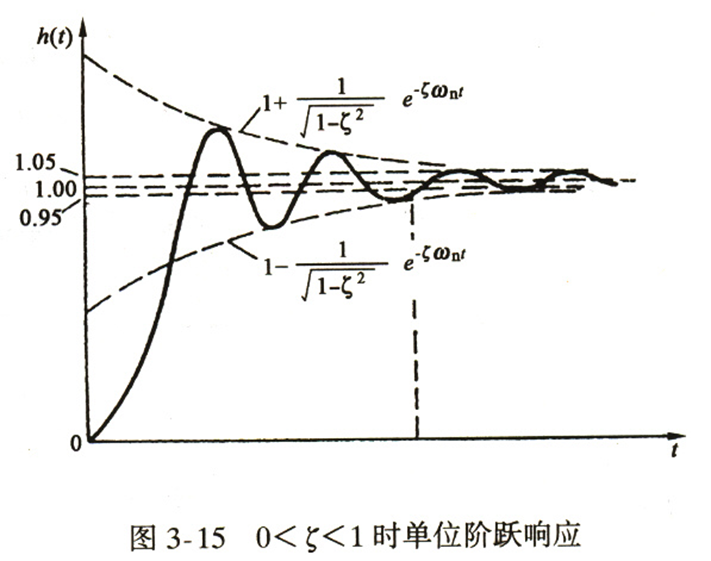两个共轭复根$\ s_{1,2}=-ζω_n±jω_n √(1-ζ^2 )$
阻尼角$\ β=tg^{-1} \frac{√(1-ζ^2 )}{ζ}$
5.总结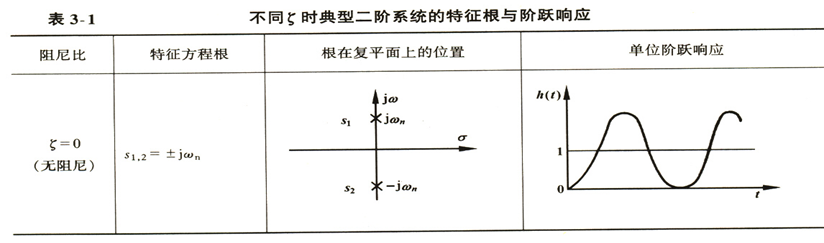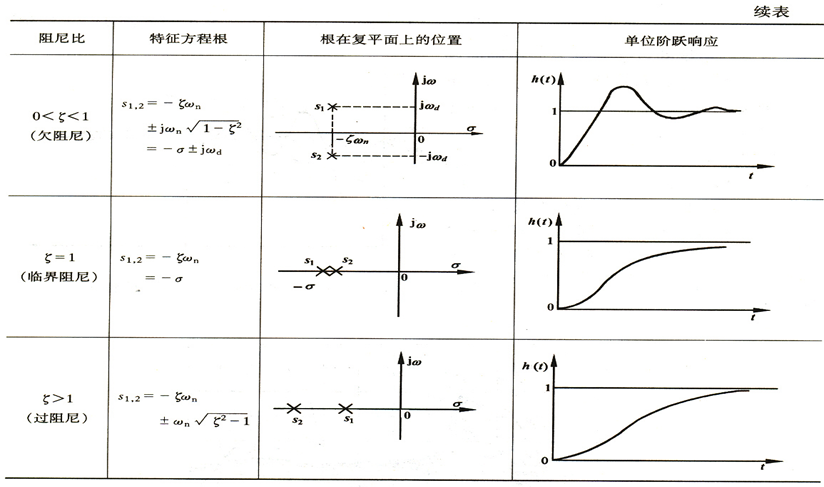三 、欠阻尼二阶系统性能指标计算
1.上升时间$\ t_r$
$\ t_r=\frac{π-β}{ω_n √(1-ζ^2 )}$
阻尼比一定时，响应速度与$\ ω_n$成正比；
阻尼振荡频率$\ ω_d$一定时，阻尼比越小，上升时间越短。
2.峰值时间$\ t_p$
$\ t_p=\frac{π}{ω_n √(1-ζ^2 )}$
峰值时间与闭环极点的虚部成反比，闭环极点离负实轴越远，峰值时间越短。
3.超调量$\ σ\%$
$\ σ\%=e^{\frac{-ζπ}{√(1-ζ^2 )}}$
超调量只与阻尼比有关，阻尼越大，超调越小。
4.调节时间$\ t_s$
$\ t_s=\frac{4}{ζω_n }$ ($\ Δ=2\%$)
或$\ t_s=\frac{3}{ζω_n }$ ($\ Δ=5\%$)
5.延迟时间$\ t_d$
当$\ 0<ζ<1$ 时，$\ t_d=\frac{1+0.7ζ}{ω_n }$
增大自然频率或减小阻尼比，可以减小延迟时间。
四 、具有零点的二阶系统分析
1、原系统条件不变时，附加一个闭环零点，将使二阶系统阶跃响应的超调量增大，上升时间和峰值时间减小
2、零点距离极点越近，上述影响越显著
3、零点距离虚轴很远时，零点影响可忽略


展开全文• 什么是控制系统的数学模型呢？顾名思义，数学模型即为用数学表达式反应出客观事物的特征与其内部的联系，而控制系统的数学模型就是描述系统动态特性与各个变量之间的关系。举个简单的例子：该系统的模型为二阶的微分...什么是控制系统的数学模型呢？顾名思义，数学模型即为用数学表达式反应出客观事物的特征与其内部的联系，而控制系统的数学模型就是描述系统动态特性与各个变量之间的关系。举个简单的例子：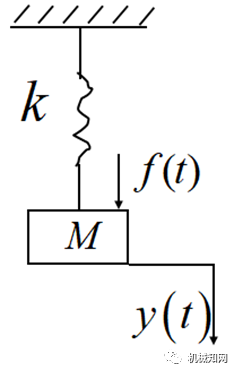该系统的模型为二阶的微分方程：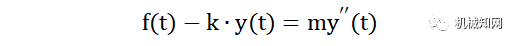先确定输入量为f(t)，输出量为y(t)则由牛顿第二定律：【注】对于这个微分方程，我们也可以这样理解：f(t)为向下的力，ky(t)为弹簧施加的向上的阻力，而m则为质量块的惯性力，它与弹簧的弹力有相同的性质：总是阻碍受力物体的运动，即施加力的方向总是与受力物体的运动方向相反，因此上式即为作用于质量块上向上的力等于向下的力。一般来说，建立元件或系统的微分方程可依据以下步骤进行：(1)确定系统或者元件的输入量、输出量。(2)按照信号的传递顺序，从系统的输入端开始，依据各变量所遵循的运动规律，列写出在运动过程中的各个环节的动态微分方程。(3)消去所列各微分方程组的中间变量，从而得到描述系统的输入、输出量的微分方程。(4)整理所得微分方程。机械系统1.机械平移系统在常见的机械平移系统的模型中，有三个基本的无源元件：质量m，弹簧k，阻尼器c。质量是一种耗能元件，主要用来吸收系统的能量，并转换成热能耗散掉，体现系统的惯性力：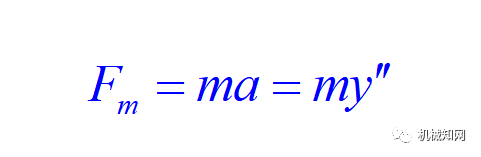弹簧属于储能元件，存储弹性势能，体现弹性力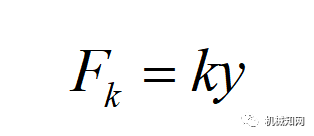阻尼器是系统中的储能元件，存储平动动能，产生粘性摩擦的阻尼力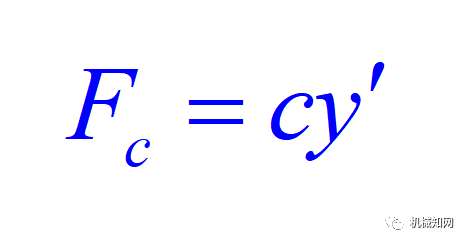在上例的基础上，增加一个阻尼器：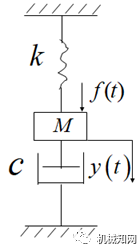1)首先确定系统的输入量和输出量：由图可知对质量块施加力f，在弹簧和阻尼器的共同作用下，系统向下产生了位移y,即f(t)为输入量，y(t)为输出量。2)整个系统遵守牛顿第二定律：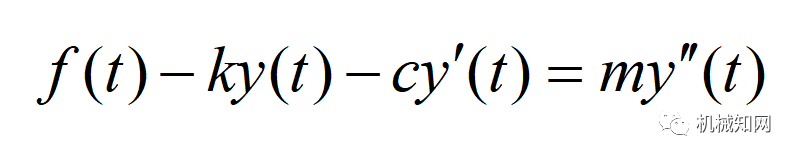3)整理后，习惯地将输出量放在等式左端，输出量放在等式右端：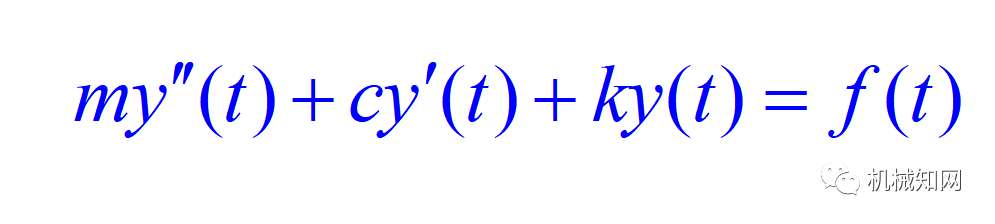上式即为该系统的控制模型，一个二阶的微分方程。在此基础上再加一个阻尼器和质量块：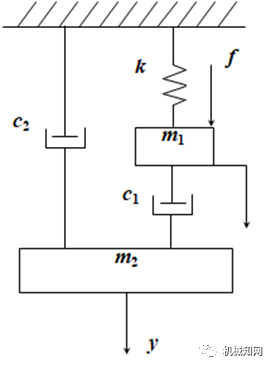1)同样，f(t)为输入量，y(t)为输出量2)此时，输入与输出之间没有必然联系，则通过假设中间变量x(t)为质量块m1的竖直位移，即可找到两者的关系：先对局部系统列写平衡方程：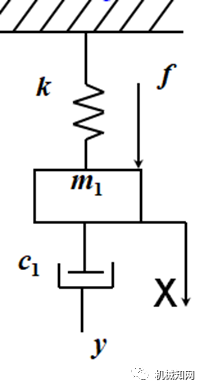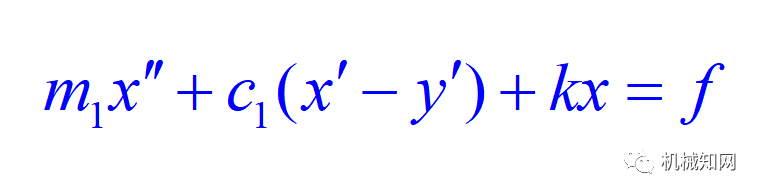再对质量块m2列写平衡方程：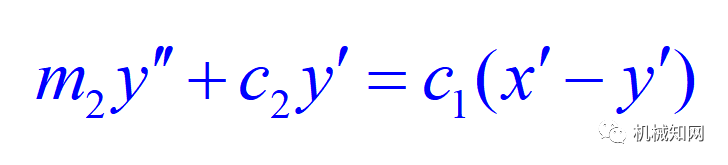联立以上两个方程，消去中间变量，整理后即为该系统的控制模型：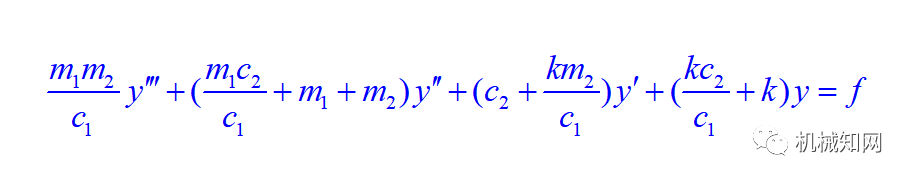机械转动系统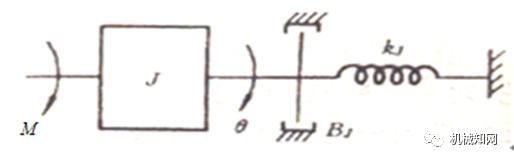回转运动所包含的要素有惯量、扭转弹簧、回转粘性阻尼。上图为在扭矩 M作用下的转动机械系统，扭矩和转角间的微分方程为：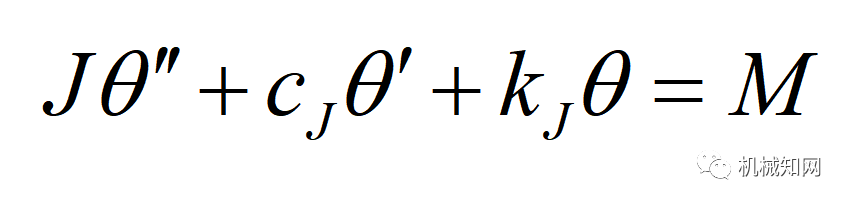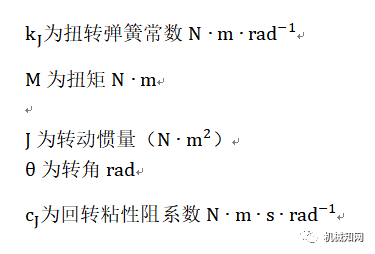以下图为例，M为输入外力矩，w为输出的角速度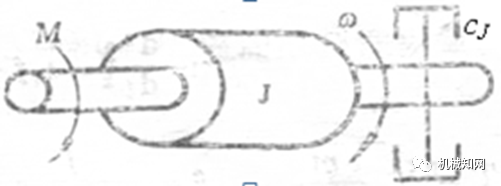则该系统的微分方程为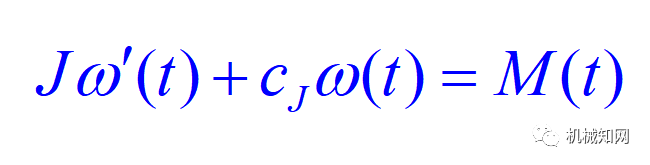当输出量为转角时，微分方程为：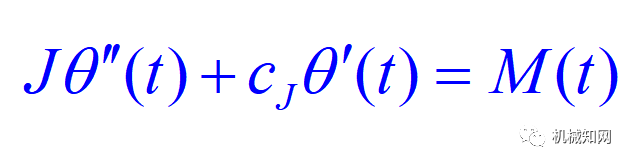RLC电网络系统电网络系统模型中，通常包含三种线性双向的无源元件：电阻R，电感L，电容C。电感是一种储存磁能的元件；   电容是储存电能的元件；电阻是一种耗能元件，  将电能转换成热能耗散掉。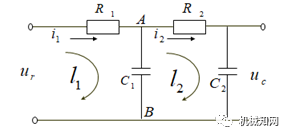该系统输入量为Ur，输出量为Uc。假设中间变量i1和i2建立输入，输出变量的关系，则对L1回路使用KVL定律：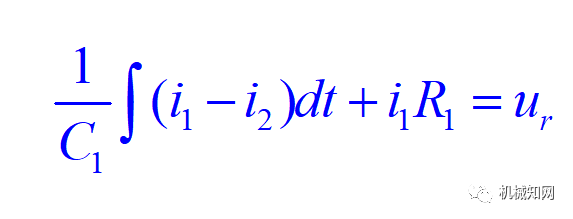对L2列写KVL方程：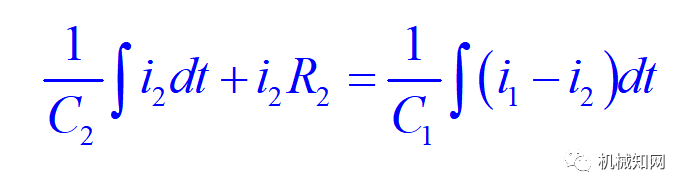且由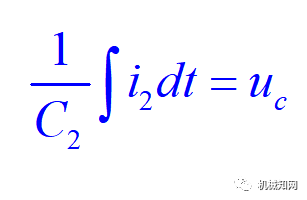消去中间变量：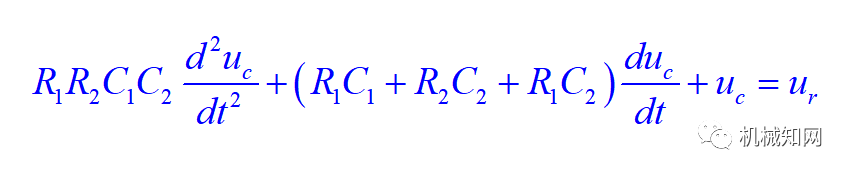☞ 内容来源：机械知网☞ 作者：知识旅途展开全文• 控制系统的数学模型背景建模方法常用的数学模型时域数学模型的建立建立微分方程的步骤复数域模型的建立传递函数的性质系统的典型环节及传递函数比例环节惯性环节积分环节微分环节理想微分环节一阶微分环节二阶微分...自动控制原理 神经网络
• 顾名思义，数学模型即为用数学表达式反应出客观事物的特征与其内部的联系，而控制系统的数学模型就是描述系统动态特性与各个变量之间的关系。举个简单的例子：该系统的模型为二阶的微分方程：先确定输入量为f(t)，...
• 对于二阶系统，可以采用解析的方法求出其时域响应，而二阶系统的分析结论有时也可以应用于高阶R 系统的分析。因此，本文将以二阶系统或二阶电路为例来分析时域特性。最简单最常用的例子是LO低通滤波器电路，忽略掉...
• 一、数学模型 二、自由振动 ... 3.4 有阻尼受迫振动（冲击响应）一、数学模型对于很多工程而言，动态特性是非常重要考核指标，其本质就是两个变量及其关系：力和位移。对于对象可以简化成质点...
• 3二阶系统的瞬态响应典型二阶系统的数学模型典型二阶系统的瞬态响应二阶系统性能指标及其系统参数的关系改善二阶系统响应特性的措施带有零点的二阶系统分析二阶系统应用广，很多高阶，复杂的系统都可以简化为二阶...
• 构建MIEGSs的数学模型，将管道方程约束松弛为线性约束，通过求解松弛模型得到管道流量初值，由初值确定管道流向；根据管道流向采用SOC松弛方法，从而避免引入整数变量，并得到区域子问题的最优解；进而采用交替方向...
• 基于模块化多电平换流器高压直流输电(MMC-HVDC)技术已得到广泛运用，但传统基于dq同步旋转坐标系双闭环PI控制中电流内环需要依赖系统数学模型进行前馈解耦补偿，并且一阶非线性自抗扰控制器设计参数过多、整定...
• 1、二阶RC数学模型的建立   一般来说，建立精准电池模型我们需要考虑以下几点：该模型能够准确描述电池动态及静态特性；该模型复杂度较低，易于计算；该模型工程实现较为简单可行。综合考虑，我们选择二阶RC...
• 首先，建立系统数学模型，通过维持第 1 杆初始状态，将系统降阶为一阶非完整系统; 其次，通过分阶段控制驱动杆角度，将系统降阶为 3 个具有完整约束两连杆子系统，并基于各子系统积分特性获得驱动杆与欠驱动杆...研究论文
• 摘要：自然界与人类社会有关系的系统绝大部分是模糊系统，这类系统的数学模型不能由经典的物理定律和数学描述来建立。本文在模糊控制理论基础上设计非最小相位系统，利用专家经验建立模糊系统控制规则库，由规则库...PID
• 锁相环数学模型 图1 锁相环复频域模型 锁相环是由鉴相器、环路滤波器、压控振荡器组成，其中系统传递函数可以表示成H(s)H(s)H(s)，误差传递函数可以表示成He(s)H_e(s)He​(s)。其中参考公式可以参考《锁相环...经验分享 算法
• 自动控制理论（2）——控制系统的数学模型（微分方程、传递函数） 自动控制理论（3）——控制系统的数学模型（系统框图和信号流图） 自动控制理论（4）——系统的时域性能指标和一阶系统的时域分析 自动控制理论（5...
• 龙格-库塔方法基本原理3.ppt 在连续系统的仿真...因此要将有些需用极限或无穷过程进行的运算有限化 对无穷过程进行截断 这样产生的误差成为截断误差 根据实际情况建立的数学模型往往难以求解 通常需要通过近似替代 ...
• 理论分析与仿真结果表明:系统服役年龄变化是一个从振荡趋于稳定的过程,可以用二阶系统的时间响应函数来描述;系统服役年龄的变化周期近似于零件服役寿命的均值,稳定值近似于振幅的平均值;对于给定的维护周期和偏差,...
• 以一个简单二阶模型介绍了非线性系统的固定时间Terminal控制器的设计方法...对于带扰动的电机模型，建立二阶数学模型。针对这一被控对象设计了Terminal终端滑模控制器，使得电机的输出在固定时间Tc时刻跟踪上期望输入。matlab
• 由物理分析得出风电机组的二阶数学模型，采用微分几何的精确反馈线性化理论，对该二阶模型进行全局线性化，得到适用于全局范围的线性模型。应用模糊控制理论对新的系统写出模糊控制规则，进而得到原系统的恒功率控制...
• 自动控制理论（2）——控制系统的数学模型（微分方程、传递函数） 自动控制理论（3）——控制系统的数学模型（系统框图和信号流图） 自动控制理论（4）——系统的时域性能指标和一阶系统的时域分析 自动控制理论（5...
• 为实现交流感应电动机高性能调速,快速跟踪变化负载转矩,对静止两相坐标系中电动机数学模型精确反馈线性化,将复杂电动机非线性系统转换成两个完全解耦线性转速和磁链二阶系统.针对解耦转速和磁链子系统...